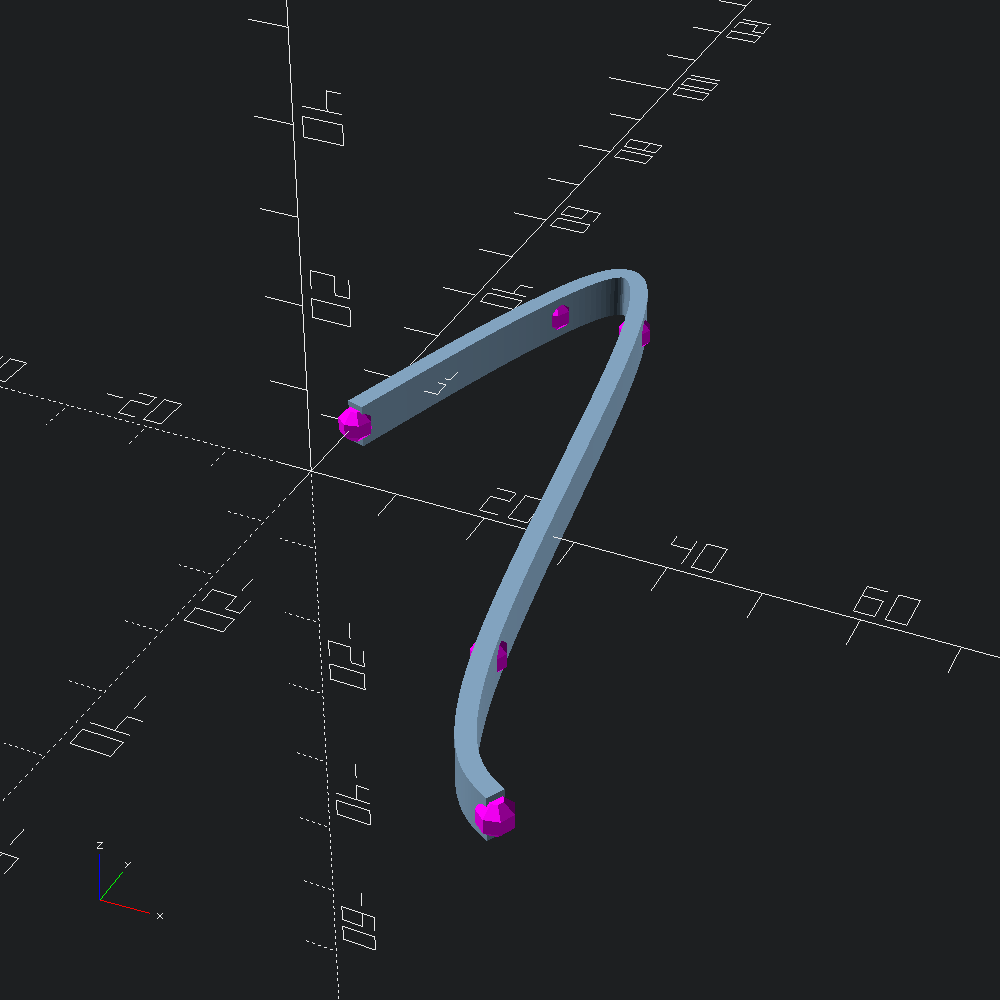# Cublic Spline

``````open OCADml

Control points that our cubic spline will pass through.

``let control = V2.[ v 0. 10.; v 10. 40.; v 20. 40.; v 30. (-20.); v 40. (-40.) ]``

Mark our `control` points with the debugging helper `Debug.show_path2` for reference. We don't really need to number these, so we'll ignore the index parameter and use the same sphere for each point.

``let marks = Debug.show_path2 Scad.(fun _ -> color Color.Magenta @@ sphere 2.) control``

Fit a cubic spline to the `control` points, and interpolate `fn` points along it using the helper `Path2.cubic_spline` (lower level functions available in the `CubicSpline` module), then sweep a `rectangle` along it.

``````let line =
let rectangle = Poly2.square ~center:true (v2 2. 5.)
and path = Path3.of_path2 @@ Path2.cubic_spline ~fn:100 control in
Union our control point `marks` and `line` sweep shapes and output to file.
``let () = Scad.to_file "spline.scad" (Scad.add line marks)``